# Professors of the Department of Differential Equations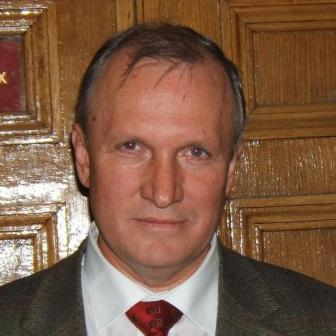Valery Vasilievich Kozlov, Academician, Chairman of the Department Research Interests: dynamical systems of the classical mechanics, integrability of differential equations, regular and chaotic dynamics, stability theory Teaching: special course on dynamical systems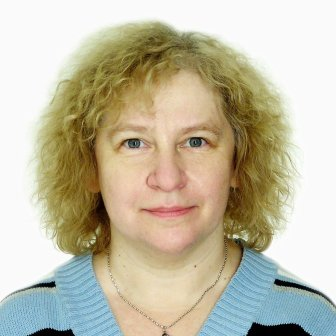Irina Victorovna Astashova, Professor, Doctor of Sciences Research Interests: qualitative theory of differential equations, asymptotic behavior of solutions Teaching: general course on differential equations, general course on partial differential equations; special course on qualitative theory of differential equations Contact e-mail: ast@diffiety.ac.ru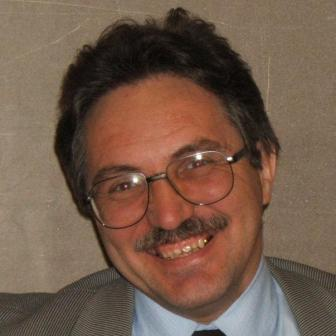Alexei Vladislavovich Borovskih, Professor, Doctor of Sciences Research Interests: partial and ordinary differential equations, integrability theory, Lie groups and algebras, wave propagation Teaching: general course on partial differential equations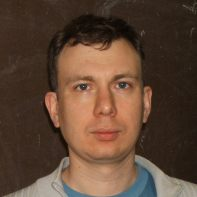Vladimir Vladislavovich Bykov, Associate Professor, PhD, Academic Secretary of the Department Research Interests: topological properties of dynamical systems, qualitative theory of ordinary differential equations Teaching: general course on differential equations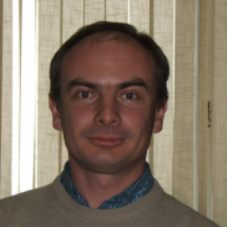Gregory Alexandrovich Chechkin, Professor, Doctor of Sciences Research Interests: partial differential equations, homogenization of solutions of differential equations, spectral theory Teaching: general course on partial differential equations; special course on the homogenization theory Contact e-mail: chechkin@mech.math.msu.su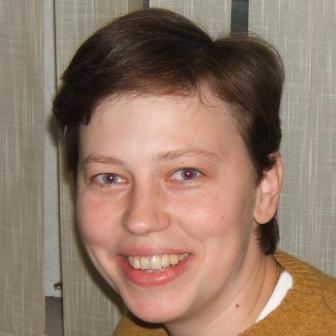Natalia Victorovna Denisova, Associate Professor, PhD Research Interests: dynamical systems, integrability problems and related issues of the qualitative theory of differential equations Teaching: general course on differential equations Contact e-mail: ndenis@mech.math.msu.su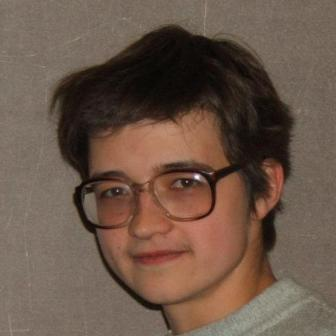Irina Vladimirovna Filimonova, Assistant Professor, PhD Research Interests: partial differential equations, asymptotic behavior of solutions of parabolic and elliptic differential equations, stabilization problem Teaching: general course on partial differential equations Contact e-mail: filimi@yandex.ru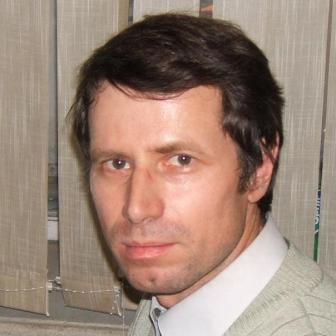Alexei Vladislavovich Filinovskii, Professor, Doctor of Sciences Research Interests: qualitative theory of partial differential equations, extremal problems, stabilization of solutions of hyperbolic equations Teaching: general course on partial differential equations Contact e-mail: flnv@yandex.ru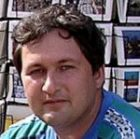Andrey Yuryevich Goritsky, Associate Professor, PhD Research Interests: infinite-dimensional dynamical systems corresponding to equations of mathematical physics, attractors, inertial and integral manifolds Teaching: general course on equations of mathematical physics; special courses "First-Order Partial Differential Equations", "Introduction to Infinite-Dimensional Dynamical Systems" Contact e-mail: goritsky@mech.math.msu.su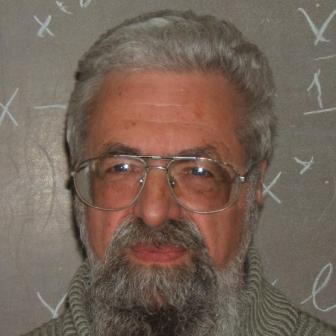Yulij Sergeyevich Ilyashenko, Professor, Doctor of Sciences Research Interests: ordinary and partial differential equations, dynamical systems, complex analysis Teaching: general course on differential equations; special courses on analytic differential equations, dynamical systems, and holomorphic dynamics; seminar "Dynamical Systems"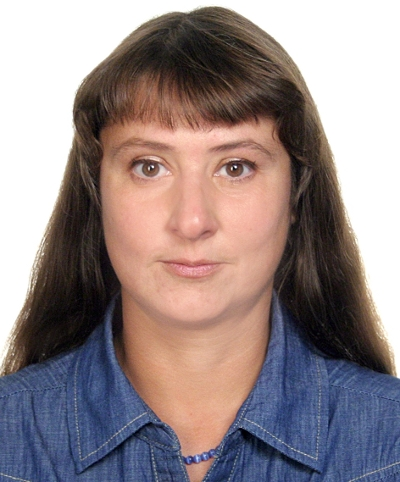Tatiana Olegovna Kapustina, Associate Professor, PhD Research Interests: partial differential equations, dinamical systems, qualitative theory of ordinary differential equations Teaching: general course on differential equations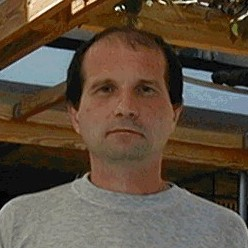Andrej Alexandrovich Kon'kov, Professor, Doctor of Sciences Research Interests: qualitative theory of partial differential equations, singular solutions and the behavior of solutions of nonlinear ordinary differential equations Teaching: general course on partial differential equations; special course on elliptic equations Contact e-mail: konkov@mech.math.msu.su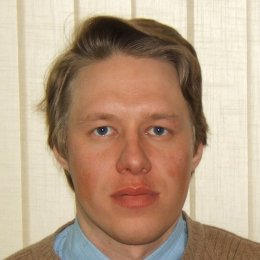Ivan Vladimirovich Matrosov, Assistant Professor, PhD Research Interests: ordinary differential equations, discontinuous differential equations, finite dimentional algebraic-differential equations, theoretical mechanics and control theory Teaching: general course on differential equations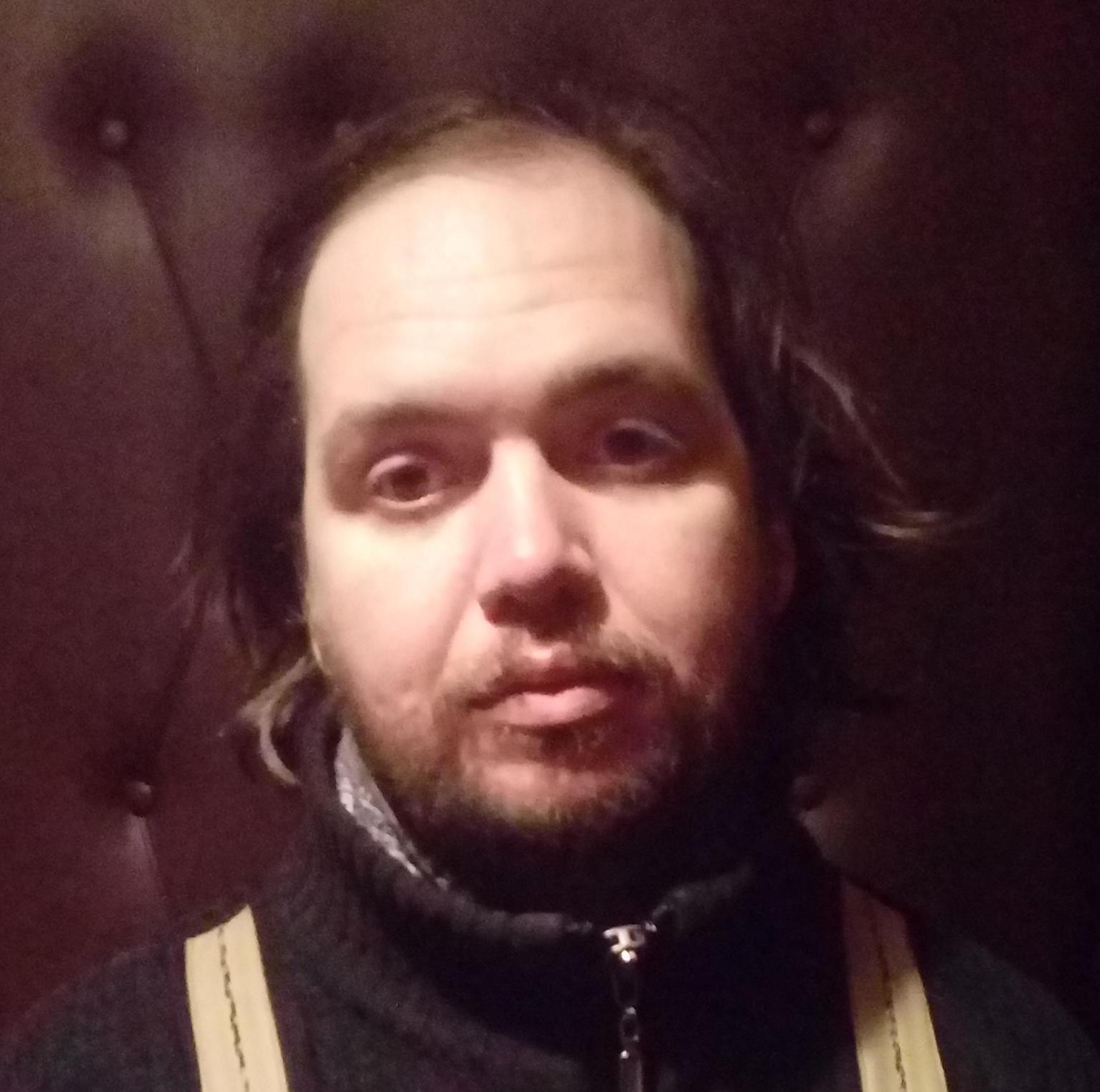Vladimir Vladimirovich Palin, Senior Lecturer, PhD Research Interests: first order partial differential equations, hyperbolic equations and systems, conservation laws Teaching: general course on partial differential equations; special cource on non-strict hyperbolic equations and systems Contact e-mail: grey_stranger84@mail.ru Contact phone: +7 (916) 844-65-97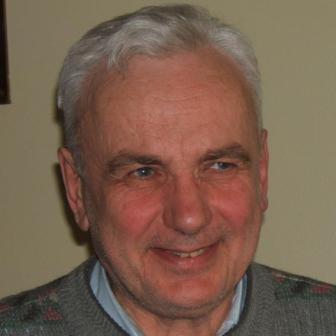Evgeniy Vladimirovich Radkevich, Professor, Doctor of Sciences Research Interests: qualitative theory of evolution boundary value problems, wave dynamics, stability of solutions of partial differential equations Teaching: general course on partial differential equations; special course on the evolution problems Contact e-mail: radk@mech.math.msu.su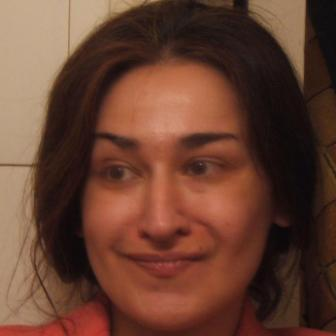Olga Sergeevna Rozanova, Professor, Doctor of Sciences Research Interests: nonlinear PDE of hyperbolic type, conservation and balance laws, nonlinear Schrodinger equation, applications to meteorology and other fields of physics, finance mathematics Teaching: general course on partial differential equations; seminar on mathematical models in economics Contact e-mail: rozanova@mech.math.msu.su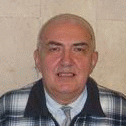Nikolai Khristovich Rozov, Professor, Doctor of Sciences Research Interests: differential equations and applications, asymptotic methods, oscillations theory, control theory Teaching: special course on differential equations; seminar on the qualitative theory of ordinary differential equations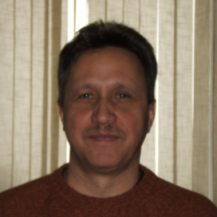Igor Nicolaevich Sergeev, Professor, Doctor of Sciences Research Interests: topological properties of dynamical systems, qualitative theory of ordinary differential equations Teaching: general course on differential equations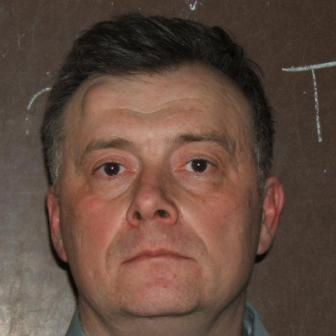Alexei Stanislavovich Shamaev, Professor, Doctor of Sciences, Vice-Chairman of the Department Research Interests: partial differential equations, control theory, stochastic differential equations, financial mathematics, asymptotic methods, wave dynamics Teaching: general course on partial differential equations; special course on stochastic differential equations Contact e-mail: shamaev@ipmnet.ru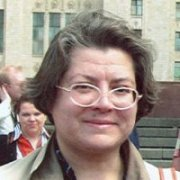Tatiana Ardolionovna Shaposhnikova, Professor, Doctor of Sciences Research Interests: partial differential equations, homogenization theory Teaching: general course on partial differential equations; special course on the homogenization theory Contact e-mail: shaposh.st@ru.net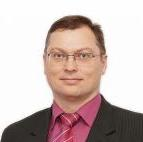Alexander Nikolaevich Vetokhin, Professor, Doctor of Sciences Research Interests: topological properties of dynamical systems, qualitative theory of ordinary differential equations, stability theory Teaching: general course on differential equations Contact e-mail: anveto27@yandex.ru